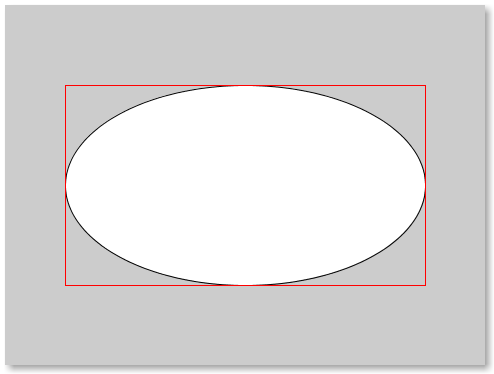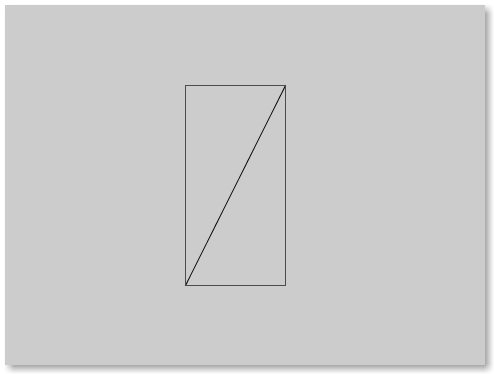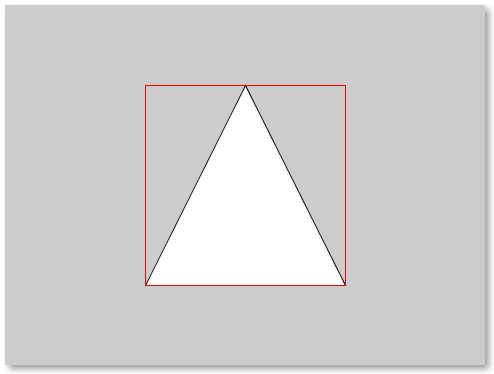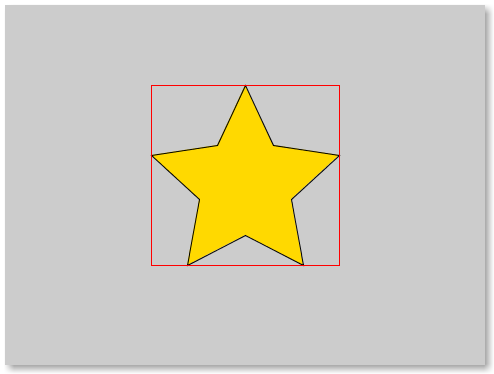# How to get bounding-box of different Shapes in p5.js ?

In this article, we will see how to get the bounding-box of different shapes. We will use P5.js which is a Javascript framework creative programming environment and is very much inspired by Processing.

Bounding-box: A bounding-box is basically just a rectangle that bounds a shape more technically it is the rectangle with the smallest possible surface area that bounds the shape. A bounding-box can have rotation (called global bounding-box) but in this article, we’ll be focusing around the axis-aligned bounding boxes (AABB shapes) which have zero rotation in them (also called local bounding-box)

Note: A Bounding-Box for a shape is the rectangle with the smallest possible surface area that bounds the shape.

Reason to calculate bounding-box: A bounding-box acts as a container of a shape and has several applications in graphical applications (most notably used by GUI libraries for widget-masks). Since a bounding-box contains the shape so if any other shape doesn’t intersect with the bounding-box then it also doesn’t intersect with the inner-shape thus bounding-boxes are used heavily in Physics engines (such as Box2D) for broad-phase collision detection.

Base for the p5.js: This is the base-code (normally for every p5.js code).

 `` `<``html``> ` `  ``<``head``> ` `    ``<``script` `src``=``"https://cdn.jsdelivr.net/npm/p5"``> ` `    ``<``script` `src``=``"sketch.js"``> ` `  `` ` `  ``<``body``> ` `  `` ` ` `

Note: We will only change script.js at every iteration, and the HTML file will necessarily remain intact!

• Finding bounding-box of an ellipse:

 `/* p5.js Sketch for finding and drawing ` `   ``bounding-box of an ellipse*/` `function` `setup(){ ` `  ``createCanvas(480, 360); ` `} ` ` `  `// Draws bounding-box around the ` `// given ellipse! ` `function` `drawBBox(x0, y0, r1, r2){ ` ` `  `  ``// Draw only the outline ` `  ``// of the rectangle ` `  ``noFill(); ` ` `  `  ``// Draw the outline in red ` `  ``stroke(255, 0, 0); ` `  ``rect(x0-r1, y0-r2, 2*r1, 2*r2); ` `} ` `function` `draw() { ` `  ``let x0 = width/2, y0 = height/2; ` `  ``let r1 = 180, r2 = 100; ` ` `  `  ``// Note that `ellipse` takes in ` `  ``// diameters not radii! ` `  ``ellipse(x0, y0, 2*r1, 2*r2); ` `  ``drawBBox(x0, y0, r1, r2); ` ` `  `  ``// We don't want to draw this ` `  ``// over and over again ` `  ``noLoop(); ` `} `

Output:• Finding bounding-box of a circle: It is same as an ellipse, since a circle is just a special case of an ellipse with same radii (same semi-major-axis and semi-minor axis).
• Finding bounding-box of a line-segment

 `/* p5.js Sketch for finding and drawing ` `  ``bounding-box of a line-segment*/` `function` `setup() { ` `  ``createCanvas(480, 360); ` `} ` ` `  `// Draws bounding-box around the ` `// given line-segment! ` `function` `drawBBox(x1, y1, x2, y2) { ` `  ``stroke(255, 0, 0); ` `  ``noFill(); ` `  ``let x = min(x1, x2), y = min(y1, y2); ` `  ``let w = max(x1, x2) - x, h = max(y1, y2) - y; ` `  ``rect(x, y, w, h); ` `} ` ` `  `function` `draw() { ` `  ``let x1 = 280, y1 = 80, x2 = 180, y2 = 280; ` `  ``line(x1, y1, x2, y2); ` `  ``drawBBox(x1, y1, x2, y2); ` `  ``noLoop(); ` `} `

Output:• Finding bounding-box of a triangle: Finding bounding-box of a triangle is very similar to finding bounding-box for line-segment.

 `/* p5.js Sketch for finding and drawing ` `  ``bounding-box of a triangle*/` `function` `setup() { ` `  ``createCanvas(480, 360); ` `} ` ` `  `// Draws bounding-box around the ` `// given triangle! ` `function` `drawBBox(x1, y1, x2, y2, x3, y3) { ` `  ``stroke(255, 0, 0); ` `  ``noFill(); ` `  ``let x = min(x1, x2, x3), y = min(y1, y2, y3); ` `  ``let w = max(x1, x2, x3) - x, h = max(y1, y2, y3) - y; ` `  ``rect(x, y, w, h); ` `} ` ` `  `function` `draw() { ` `  ``let x1 = 240, y1 = 80, x2 = 140; ` `  ``let y2 = 280, x3 = 340, y3 = 280; ` ` `  `  ``triangle(x1, y1, x2, y2, x3, y3); ` `  ``drawBBox(x1, y1, x2, y2, x3, y3); ` `  ``noLoop(); ` `} `

Output:• Finding bounding-box of a polygon: A triangle is a polygon, and if we find the bounding-box of a triangle then finding bounding-box for polygon shouldn’t be any difficulty. We just have to generalize so that we can have any number of vertices and we are done.

 `/* p5.js sketch for finding and drawing ` `  ``bounding-box of a polygon*/` `function` `setup() { ` `  ``createCanvas(480, 360); ` `} ` ` `  `// Draws bounding-box around ` `// the given polygon! ` `function` `drawBBox(x, y) { ` `  ``stroke(255, 0, 0); ` `  ``noFill(); ` `  ``let rx = min(x), ry = min(y); ` `  ``let w = max(x) - rx, h = max(y) - ry; ` `  ``rect(rx, ry, w, h); ` `} ` ` `  `function` `draw(){ ` `  ``/* Vertices for a star-polygon (decagon) */` `  ``let x = [240, 268, 334, 286, 298, ` `           ``240, 182, 194, 146, 212]; ` `  ``let y = [80, 140, 150, 194, 260, ` `           ``230, 260, 194, 150, 140]; ` ` `  `  ``beginShape(); ` ` `  `  ``for` `(let i = 0; i < x.length; ++i) ` `    ``vertex(x[i], y[i]); ` `  ``fill(255, 217, 0); ` ` `  `  ``// If you don't CLOSE it then it'd ` `  ``// draw a chained line-segment ` `  ``endShape(CLOSE); ` `  ``drawBBox(x, y); ` `  ``noLoop(); ` `} `

Output:Finding Bounding-Boxes is an important part of visualization applications. Also in dynamic applications such as games, one cannot compute capsule-collision detection at every frame without entailing a punishment in the performance. So before any complex collision-checking, a broad-phase check is made for early exit which returns false as soon as it is ascertained that the shape doesn’t collide with the other shape. If the broad-phase check is passed then comes the narrow-phase where the actual collision-detection (OOBB, SAT, capsule, ellipsoid, etc) happens! Hence finding the bounding-box is an important part of many graphics-rich applications for various reasons.My Personal Notes arrow_drop_upCheck out this Author's contributed articles.

If you like GeeksforGeeks and would like to contribute, you can also write an article using contribute.geeksforgeeks.org or mail your article to contribute@geeksforgeeks.org. See your article appearing on the GeeksforGeeks main page and help other Geeks.

Please Improve this article if you find anything incorrect by clicking on the "Improve Article" button below.

1

Please write to us at contribute@geeksforgeeks.org to report any issue with the above content.